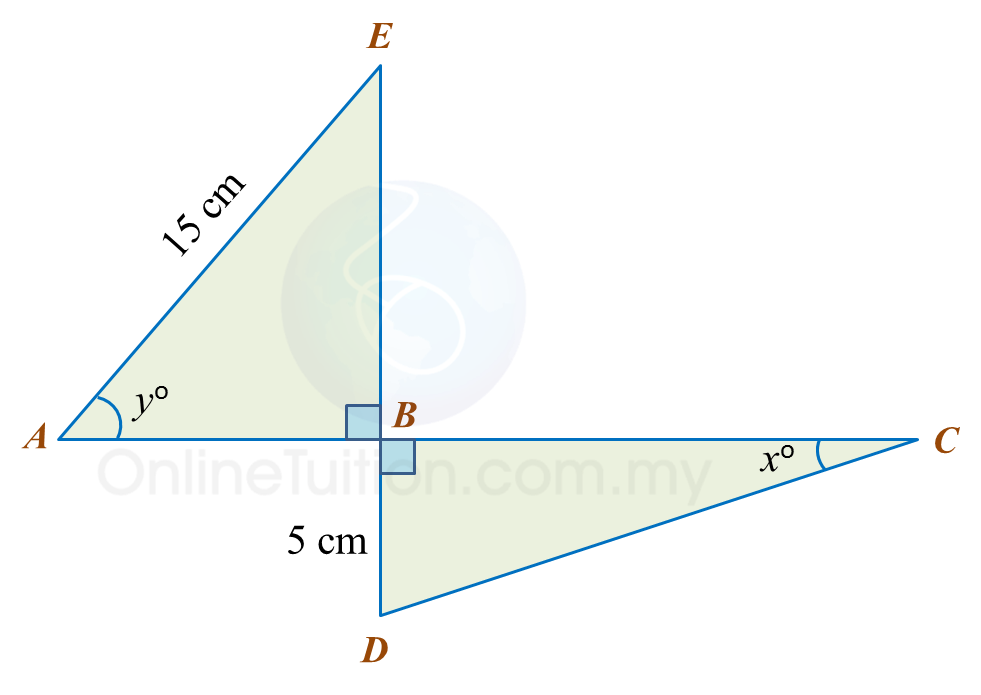# 15.2.1 Trigonometry, PT3 Focus Practice

 
 
15.2.1 Trigonometry, PT3 Focus Practice
 

 
Question 1:
 
Diagram below shows a right-angled triangle ABC.It is given that    $\mathrm{cos}{x}^{o}=\frac{5}{13}$   , calculate the length, in cm, of AB.
 
 

Solution:
     

 
 
 
 
 
Question 2:
 
In the diagram, PQR and QTS are straight lines.It is given that $\mathrm{tan}y=\frac{3}{4}$ , calculate the length, in cm, of RS.
 
 

Solution:
     

 
 
 
 
 
Question 3:
 
In the diagram, PQR is a straight line.It is given that   $\mathrm{cos}{x}^{o}=\frac{3}{5}$  , hence sin yo =
 
 
 
 
Solution:
 
 

 
 
 

 
 
 
 
 
Question 4:
 
Diagram below consists of two right-angled triangles.Determine the value of cos xo.
 
 

Solution:
     
 

 
 
 
 
 
Question 5:
 
Diagram below consists of two right-angled triangles ABE and DBC.
 
ABC and EBD are straight lines.It is given that
 
(a)  Find the value of tan xo.
 
(b)   Calculate the length, in cm, of ABC.
 
 

Solution:
 
(a)
 
   
 
 
 
(b)